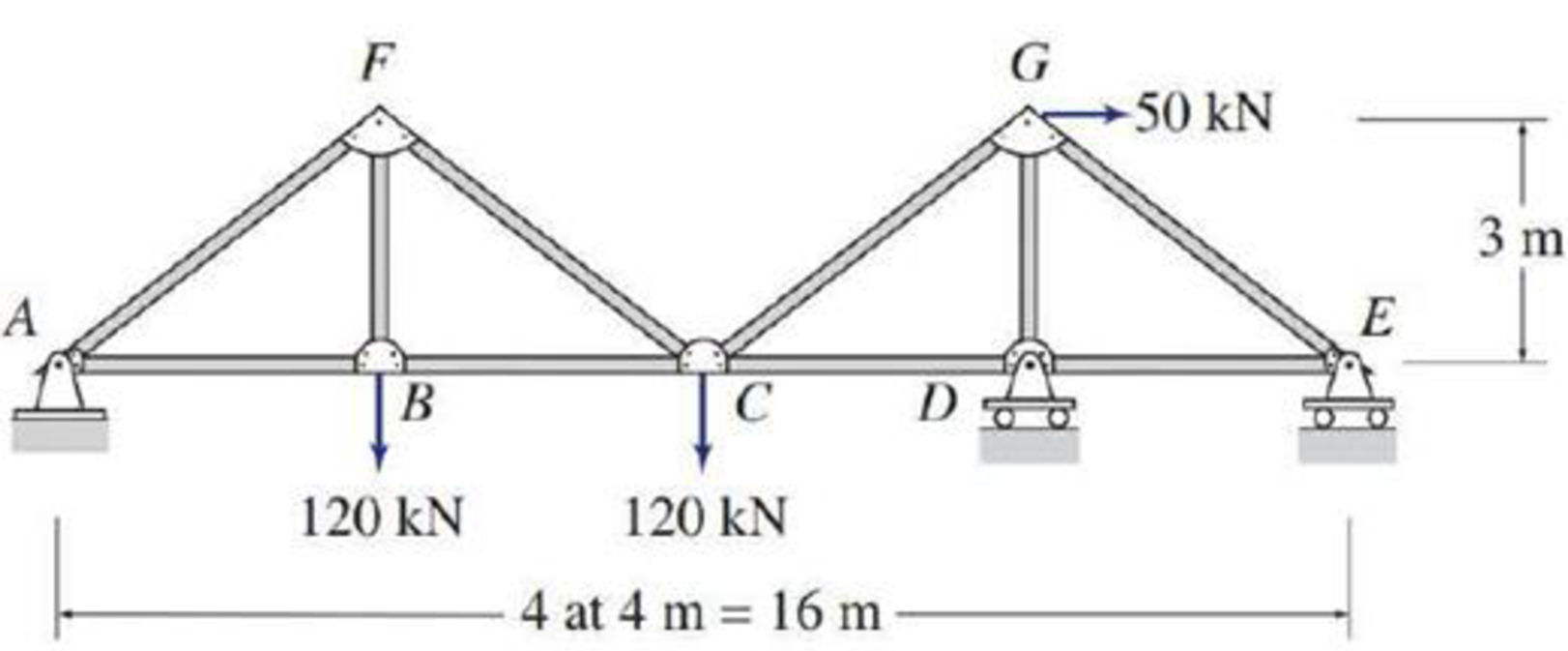# 4.6 through 4.28 Determine the force in each member of the truss shown by the method of joints. FIG. P4.28

#### Solutions

Chapter
Section
Chapter 4, Problem 28P
Textbook Problem
71 views

## 4.6 through 4.28 Determine the force in each member of the truss shown by the method of joints.FIG. P4.28

To determine

Find the forces in the members of the truss by the method of joints.

### Explanation of Solution

Given information:

Apply the sign conventions for calculating reactions, forces and moments using the three equations of equilibrium as shown below.

• For summation of forces along x-direction is equal to zero (Fx=0), consider the forces acting towards right side as positive (+) and the forces acting towards left side as negative ().
• For summation of forces along y-direction is equal to zero (Fy=0), consider the upward force as positive (+) and the downward force as negative ().
• For summation of moment about a point is equal to zero (Matapoint=0), consider the clockwise moment as negative and the counter clockwise moment as positive.

Method of joints:

The negative value of force in any member indicates compression (C) and the positive value of force in any member indicates Tension (T).

Calculation:

Consider the forces in the member AB, BC, CD, DE, BF, DG, AF, CF, CG, and EG are FAB,FBC,FCD,FDE,FBF,FDG,FAF,FCF,FCG,FEG.

Show the free body diagram of the truss as shown in Figure 1.

Refer Figure 1.

Consider the horizontal and vertical reactions at A are Ax and Ay.

Consider the vertical reaction at D and E are Dy and Ey.

Find the value of the angle θ as follows:

tanθ=34θ=tan1(34)θ=36.869°

Take the sum of the forces in the horizontal direction as zero.

Fx=0Ax+50=0Ax=50kN

Consider the member AC.

Take the sum of the moments at C is zero. Then,

MCAC=0(Ay×8)+(120×4)=08Ay=480Ay=(4808)Ay=60kN

Take the sum of the moments at E is zero. Then,

ME=0(Ay×16)+(120×12)+(120×8)(50×3)Dy×4=016Ay+1440+9604Dy150=016Ay+24004Dy150=0

16Ay+24004Dy150=0Dy=16×60+24001504Dy=12904Dy=322.5kN

Take the sum of the forces in the vertical direction is zero.

Fy=0Ay+Dy+Ey120120=0(60)+(322.5)+Ey240=0Ey=142.5kN

Show the joint A as shown in Figure 2.

Refer Figure 2.

Find the forces in the members AF and AB.

For the Equilibrium of forces,

Fy=0FAFsin(36.869°)+Ay=0FAFsin(36.869°)=AyFAF=60sin(36.869°)FAF=100kNFAF=100kN(C)

Fx=0FAFcos(36.869°)+FAB+Ax=0100cos(36.869°)+FAB+(50)=0FAB=100cos(36.869°)+50FAB=130kN(T)

Show the joint B as shown in Figure 3.

Refer Figure 3.

Find the force in the member BC and BF as follows:

For the Equilibrium of forces,

Fy=0FBF120=0FBF=120kN(T)

Fx=0FAB+FBC=0FBC=FABFBC=130kN(T)

Show the joint E as shown in Figure 4

### Still sussing out bartleby?

Check out a sample textbook solution.

See a sample solution

#### The Solution to Your Study Problems

Bartleby provides explanations to thousands of textbook problems written by our experts, many with advanced degrees!

Get Started

Find more solutions based on key concepts
For the cantilever beam relationship given in Problem 6.21, if the units of y, w, E, x, and L are expressed in ...

Engineering Fundamentals: An Introduction to Engineering (MindTap Course List)

Explain why graph databases tend to struggle with scaling out.

Database Systems: Design, Implementation, & Management

Is batch processing still relevant? Why or why not?

Systems Analysis and Design (Shelly Cashman Series) (MindTap Course List)

Define the terms, thumbnail and infographic.

Enhanced Discovering Computers 2017 (Shelly Cashman Series) (MindTap Course List)

How does a signature-based IDPS differ from a behavior-based IDPS?

Principles of Information Security (MindTap Course List)

What is middleware?

Fundamentals of Information Systems

If your motherboard supports ECC DDR3 memory, can you substitute non-ECC DDR3 memory?

A+ Guide to Hardware (Standalone Book) (MindTap Course List)

Why is the oxygen valve turned on before starting to clean a cutting tip?

Welding: Principles and Applications (MindTap Course List)